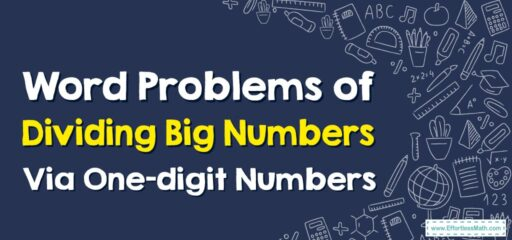# How to Solve Word Problems of Dividing Big Numbers

Word problems involving dividing big numbers by one-digit numbers require applying division concepts to real-life situations.## A Step-by-step Guide to Solving Word Problems of Dividing Big Numbers Via One-digit Numbers

Here’s a step-by-step guide to solving word problems that involve dividing big numbers by one-digit numbers:

### Step 1: Read the word problem carefully

Understand the given information, identify the numbers involved, and determine what the problem is asking you to find.

### Step 2: Identify the dividend and divisor

The dividend is the large number being divided, and the divisor is the one-digit number that represents the grouping or sharing size.

### Step 3: Set up the division problem

Write the dividend on the left side and the divisor on the outside. Leave space for the quotient.

### Step 4: Divide the first digit

Divide the leftmost digit of the dividend by the divisor. Write the quotient above the line. If the digit is smaller than the divisor, bring down the next digit to the right of it.

### Step 5: Multiply and subtract

Multiply the quotient from the previous step by the divisor and subtract the result from the digits you brought down. Write the new result below the line.

### Step 6: Repeat the division process

Repeat steps 4 and 5 for the next digit of the dividend, bringing down the next digit as needed.

### Step 7: Continue until you have divided all the digits

Repeat steps 4 and 5 for each subsequent digit of the dividend until you have divided all the digits.

### Step 8: Check your work

Multiply the quotient by the divisor and add any remainder. Ensure that the product matches the original dividend.

### Step 9: Answer the question in the word problem

Use the quotient to answer the question posed in the problem. Make sure to provide a relevant and meaningful answer.

### Step 10: Write the final answer

Express your answer in a complete sentence that relates to the context of the problem.

By following these steps, you can effectively solve word problems that involve dividing big numbers by one-digit numbers. It’s important to carefully understand the problem, set up the division correctly, and perform the necessary calculations to find the solution.

### What people say about "How to Solve Word Problems of Dividing Big Numbers - Effortless Math: We Help Students Learn to LOVE Mathematics"?

No one replied yet.

X
30% OFF

Limited time only!

Save Over 30%

SAVE $5 It was$16.99 now it is \$11.99